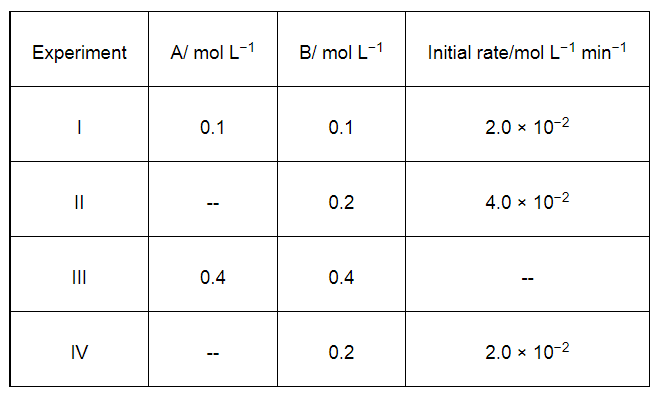# The reaction between A and B is first order with respect to A and zero order with respect to B.

Question:

The reaction between A and B is first order with respect to A and zero order with respect to B. Fill in the blanks in the following table:Solution:

The given reaction is of the first order with respect to A and of zero order with respect to B.

Therefore, the rate of the reaction is given by,

Rate = [A]1 [B]0

⇒ Rate = [A]

From experiment I, we obtain

2.0 × 10−2 mol L−1 min−1 = k (0.1 mol L−1)

⇒ k = 0.2 min−1

From experiment II, we obtain

4.0 × 10−2 mol L−1 min−1 = 0.2 min−1 [A]

⇒ [A] = 0.2 mol L−1

From experiment III, we obtain

Rate = 0.2 min−1 × 0.4 mol L−1

= 0.08 mol L−1 min−1

From experiment IV, we obtain

2.0 × 10−2 mol L−1 min−1 = 0.2 min−1 [A]

⇒ [A] = 0.1 mol L−1